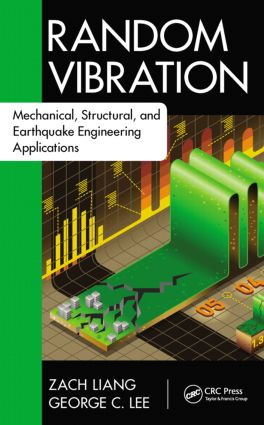# Random Vibration

## Mechanical, Structural, and Earthquake Engineering Applications, 1st Edition

CRC Press

668 pages | 192 B/W Illus.

##### Purchasing Options:\$ = USD
Hardback: 9781498702348
pub: 2015-04-14
SAVE ~\$31.00
\$155.00
\$124.00
x
eBook (VitalSource) : 9780429183539
pub: 2015-04-14
from \$77.50

FREE Standard Shipping!

### Description

Focuses on the Basic Methodologies Needed to Handle Random Processes

After determining that most textbooks on random vibrations are mathematically intensive and often too difficult for students to fully digest in a single course, the authors of Random Vibration: Mechanical, Structural, and Earthquake Engineering Applications decided to revise the current standard. This text incorporates more than 20 years of research on formulating bridge design limit states. Utilizing the authors’ experience in formulating real-world failure probability-based engineering design criteria, and their discovery of relevant examples using the basic ideas and principles of random processes, the text effectively helps students readily grasp the essential concepts. It eliminates the rigorous math-intensive logic training applied in the past, greatly reduces the random process aspect, and works to change a knowledge-based course approach into a methodology-based course approach. This approach underlies the book throughout, and students are taught the fundamental methodologies of accounting for random data and random processes as well as how to apply them in engineering practice.

Gain a Deeper Understanding of the Randomness in Sequences

Presented in four sections, the material discusses the scope of random processes, provides an overview of random processes, highlights random vibrations, and details the application of the methodology. Relevant engineering examples, included throughout the text, equip readers with the ability to make measurements and observations, understand basic steps, validate the accuracy of dynamic analyses, and master and apply newly developed knowledge in random vibrations and corresponding system reliabilities.

Comprising 11 Chapters, this text:

• Reviews the theory of probability and applies it from an engineering perspective
• Introduces basic concepts and formulas to prepare for discussions of random processes
• Emphasizes the essence of probability as the chance of occurrence in sample space
• Covers two important issues in engineering practice, the uncertainty of data and the probability of failure
• Explores the random processes in the time domain
• Explains the nature of time-varying variables by joint PDF through the Kolmogorov extension
• Examines random processes in the frequency domain
• Discusses several basic and useful models of random processes
• Presents a new set of statistics for random processes
• Employs an approach to present important processes within the context of practical engineering problems
• Includes the generality of dealing with randomness and the difference between random variables and processes
• Focuses on the topic of vibration problems
• Addresses the basic parameters of linear single-degree-of-freedom (SDOF) systems
• Stresses a new method of random process referred to as time series
• Details linear multi-degree-of-freedom (MDOF) systems
• Describes the statistical analyses of direct approach based on model decoupling of proportionally and nonproportionally-damped systems
• Provides materials on the applications of random processes and vibration
• Discusses statistical studies on random data and model identifications
• Describes the nonlinear phenomena and the general approach of linearization
• Highlights a special method of Monte Carlo simulation, and more

Random Vibration: Mechanical, Structural, and Earthquake Engineering Applications effectively integrates the basic ideas, concepts, principles, and theories of random processes. This enables students to understand the basic methodology and establish their own logic to systematically handle the issues facing the theory and application of random vibrations.

### Reviews

"Random vibration is an important branch in [the] area of dynamic structure. At the same time, it is an obscure portion to most engineering students and researchers. In this book, the author present[s] a clear method to resolve this difficulty, by showing lots of real examples or practical engineering following the basic concept about stochastic process. It constructs a bridge between these two parts instead of basing rigorous mathematical logic creatively."

—Professor Baitao Sun, Institute of Engineering Mechanics, China Earthquake Administration, Harbin

"… an excellent resource for students to learn basic methodologies handling random processes and a great reference material for researchers exploring their research on principles of multiple hazard design for structures. … admirable for its content … The authors successfully make a breakthrough that avoids mathematical difficulties for students to absorb the essential concepts needed to account for random data and processes, and effectively help readers apply the methodologies to solve their problems. … exceptional material for the study of random vibration."

—Kuo-Chun Chang, Professor, Department of Civil ngineering, National Taiwan University, Director, National Center for Research on Earthquake Engineering, Taipei, Taiwan

### Table of Contents

Section I Basic Probability Theory

Introduction

Background of Random Vibration

Fundamental Concept of Probability Theory

Random Variables

Functions of Random Variables

Systems and Functions

Sums of Random Variables

Other Functions of Random Variables

Design Considerations

Central Limit Theorems and Applications

Section II Random Process

Random Processes in the Time Domain

Definitions and Basic Concepts

Stationary Process

Correlation Analysis

Random Processes in the Frequency Domain

Spectral Density Function

Spectral Analysis

Practical Issues of PSD Functions

Spectral Presentation of Random Process

Statistical Properties of Random Process

Level Crossings

Extrema

Accumulative Damages

Section III Vibrations

Single-Degree-of-Freedom Vibration Systems

Concept of Vibration

Periodically Forced Vibration

Response of SDOF System to Arbitrary Forces

Response of SDOF Linear Systems to Random Excitations

Stationary Excitations

White Noise Process

Engineering Examples

Coherence Analyses

Time Series Analysis

Random Vibration of MDOF Linear Systems

Modeling

Direct Model for Determining Responses

Normal Mode Method

Nonproportionally Damped Systems, Complex Modes

Modal Combination

Section IV Applications and Further Discussions

Inverse Problems

Introduction to Inverse Problems

System Parameter Identification

Vibration Testing

Failures of Systems

3σ Criterion

First Passage Failure

Fatigue

Considerations on Reliability Design

Nonlinear Vibrations and Statistical Linearization

Nonlinear Systems

Nonlinear Random Vibrations

Monte Carlo Simulations

References

Index

### Subject Categories

##### BISAC Subject Codes/Headings:
TEC009070
TECHNOLOGY & ENGINEERING / Mechanical
TEC009120
TECHNOLOGY & ENGINEERING / Civil / Earthquake
TEC063000
TECHNOLOGY & ENGINEERING / Structural